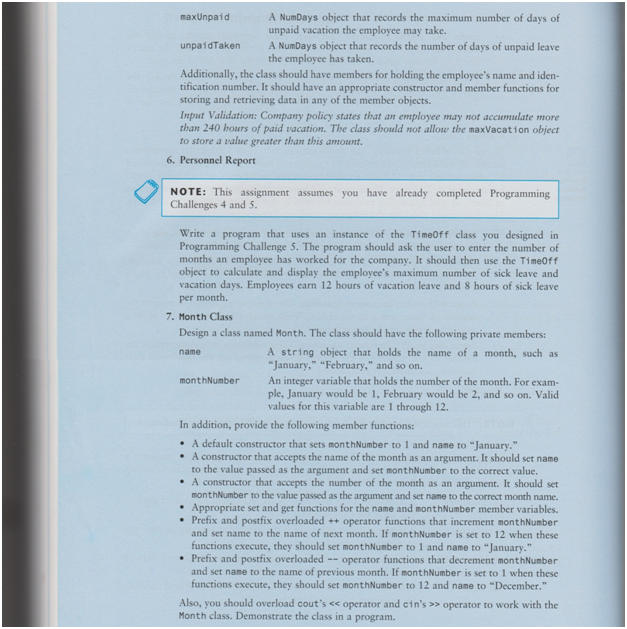# Using C++ to create a simple flowchart

Creating flowcharts using C++ can be a little confusing especially for students who are just getting started with this programming language. We provide help with this concept to make its basics understandable for students.

## Simple Flowchart in C++

Develop a flowchart and then write a C++ program to solve the following program.
Upon execution of the program, your name will be displayed, properly centered. This is not a block letters asin H2. The name is your real name like John Doe. You will be using the logical operators, relational operators and selection structures for thisassignment.
The program will then prompt the user for two integer inputs and the program will then compute the sumand the product of the two numbers and produce the results of both operations with appropriate labels. Seesample output for the addition operation below. The output assumes a = 5, and b = 10.
Sum of a and b is equal to a + b or 5 + 10 and the result is 15.
If the result is true, display true. If it is not true, display false.
The format for the product of the two numbers is the same.
The program will then use the same two integer numbers and perform the following operations. Theprogram displays the relations between the two numbers for the following relational operators:
>=, ==, <
Each of these operators requires two operands: use the first integer number as the first operand and thesecond integer number as the second operand. See sample of output desired below.
a < b is 5 < 10 and the result is true
If the result is true, display true. If it is not true, display false.
where a is the first number and b is the second number. Your solution will work for any two integernumbers.
The format for other operators listed is the same for these operations.
The program will then use the same two integer numbers and computes different operations using thefollowing arithmetic assignment operators:
‐=, /=, %=
The result of these operations is stored in the first operand. See example below.
a ‐= b is 5 ‐= 10 and the result is a = ‐5
If the result is true, display true. If it is not true, display false.
or
a /= b is ‐5 /= 10 and the result is a = 0
If the result is true, display true. If it is not true, display false.
Note that a new value of a is used after each operation (i.e. a is rippled to other operations).
The format for other operators listed is the same.
Hints:
Your program will only have one main() function, one return statement, and anything declared globally,
like #include . Parts b, c, and d are part of one .cpp file.
2. Make sure your program executes correctly
3. Make sure the variables are correctly declared without any conflicts with other variables. You may have tochange some of the variables to make each variable unique.
4. You need to run the program four times for the numbers shown below.
5. Use conditional constructs or selection structures (like if, if‐else, etc) for this assignment.

#### Solution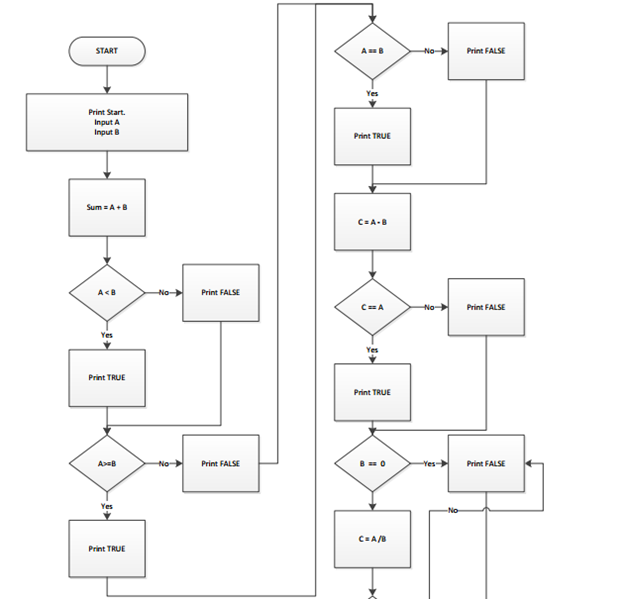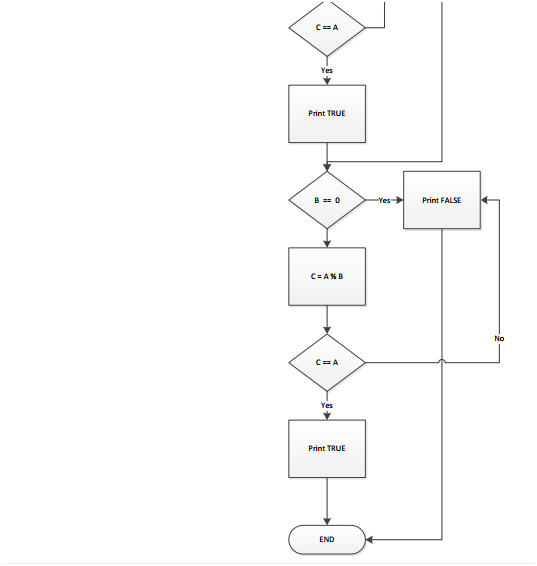#include

using namespace std;

int main(int argc, char* argv[])

{

int firstNumber, secondNumber;

cout << “Home Work 3 – ” << endl;

cout << “Enter first number: “;

cin >> firstNumber;

cout << “Enter second number: “;

cin >> secondNumber;

// Sum

cout << “Sum of a and b is equal to a + b or ” << firstNumber << ” + ” << secondNumber << ” and the result is ” << firstNumber + secondNumber << endl;

// <

cout << “a < b is ” << firstNumber << ” < ” << secondNumber << ” and the result is “;

if (firstNumber < secondNumber)

{

cout << “TRUE”;

}

else

{

cout << “FALSE”;

}

cout << endl;

// >=

cout << “a >= b is ” << firstNumber << ” >= ” << secondNumber << ” and the result is “;

if (firstNumber >= secondNumber)

{

cout << “TRUE”;

}

else

{

cout << “FALSE”;

}

cout << endl;

// ==

cout << “a == b is ” << firstNumber << ” == ” << secondNumber << ” and the result is “;

if (firstNumber == secondNumber)

{

cout << “TRUE”;

}

else

{

cout << “FALSE”;

}

cout << endl;

//-=

int result = firstNumber – secondNumber;

cout << “a -= b is ” << firstNumber << ” -= ” << secondNumber << ” and the result is a = ” << result << “: “;

if (firstNumber == result)

{

cout << “TRUE”;

}

else

{

cout << “FALSE”;

}

cout << endl;

// /=

if (secondNumber == 0)

{

cout << “a /= b is ” << firstNumber << ” /= ” << secondNumber << “: Can NOT devide for Zero FALSE” << endl;

}

else

{

result = firstNumber / secondNumber;

cout << “a /= b is ” << firstNumber << ” /= ” << secondNumber << ” and the result is a = ” << result << “: “;

if (firstNumber == result)

{

cout << “TRUE”;

}

else

{

cout << “FALSE”;

}

cout << endl;

}

// %=

if (secondNumber == 0)

{

cout << “a %= b is ” << firstNumber << ” %= ” << secondNumber << “: Can NOT devide for Zero FALSE” << endl;

}

else

{

result = firstNumber % secondNumber;

cout << “a %= b is ” << firstNumber << ” %= ” << secondNumber << ” and the result is a = ” << result << “: “;

if (firstNumber == result)

{

cout << “TRUE”;

}

else

{

cout << “FALSE”;

}

cout << endl;

}

system(“pause”);

return 0;

}

Capture.PNG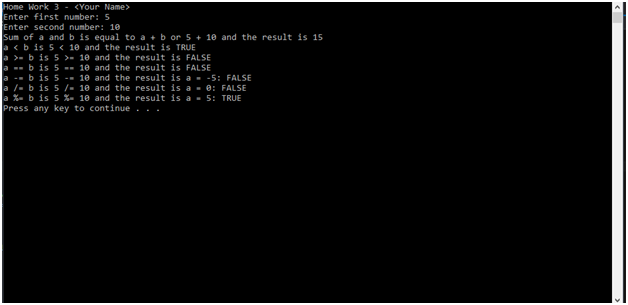Capture1.PNG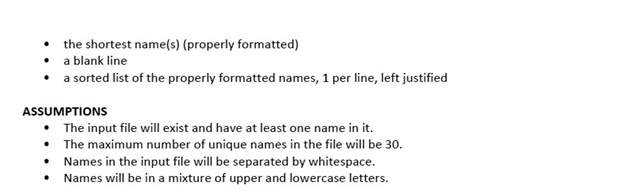Capture2.PNG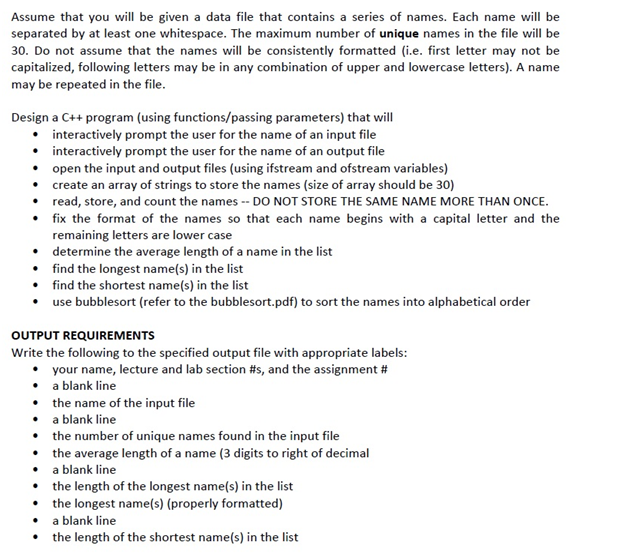Capture3.PNG